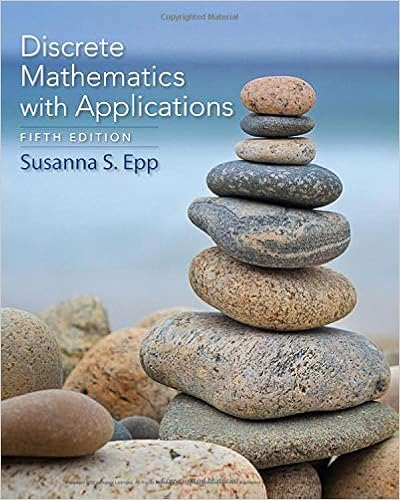# B what is the probability that ron added juniper

• Test Prep
• 8
• 100% (6) 6 out of 6 people found this document helpful

This preview shows page 7 - 8 out of 8 pages.

##### We have textbook solutions for you!
The document you are viewing contains questions related to this textbook.The document you are viewing contains questions related to this textbook.
Chapter 9 / Exercise 2
Discrete Mathematics With Applications
EppExpert Verified
b)What is the probability that Ron added juniper berries and the potion did not work? (3 points)
c)What is the probability that the potion worked? (3 points)
d)If the potion did not work, what is the probability that Ron added mistletoe berries? (3 points)
e)Is whether the potion worked independent of what type of berry Ron added? Answer YES or NO, and show mathematically why or why not. (4 points)
##### We have textbook solutions for you!
The document you are viewing contains questions related to this textbook.The document you are viewing contains questions related to this textbook.
Chapter 9 / Exercise 2
Discrete Mathematics With Applications
EppExpert Verified
8 12.Sophie will play a game in which she will flip a fair coin twice, then draw a card from a pile. There are 3 cards in the pile. Each card has one of the following numbers---1, 2, or 3 (all three cards are different). Assume all possible outcomes are equally likely. (Note: in flipping a coin there are two outcomes because there are two sides of a cointhese two are commonly called Heads and Tails.)a)Write out the sample space for this game. (For example one outcome is HT2heads, then tails, then a 2.) (4 points) S = {HH1, HH2, HH3, HT1, HT2, HT3, TH1, TH2, TH3, TT1, TT2, TT3} FOR parts b)through e)--Define the following events: ?: at least one Tail
b)Find 𝑃(?) (2 points)
c)Find𝑃(?𝐶)(2 points)
4
d)Find 𝑃(?|?)(3 points)
e)Find 𝑃((?𝐶∩ ?)𝐶).(3 points) can use DeMorgen’s to simplify𝑃((?𝐶∩ ?)𝐶) = 𝑃(? ∪?𝐶) = 1112or ? ∪?
f)Sophie wins the game if she gets tails twice or a 3 on her turn. What is the probability that Sophie wins? (3 points)
•••